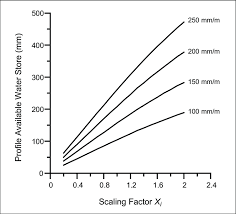## How to Calculate and Solve for Depth of Available Water | Irrigation Water RequirementThe image above represents depth of available water.

To compute for depth of available water, three essential parameters are needed and these parameters are Apparent Specific Gravity of the Soil (s), Depth of the Root Zone of the Plant (d) and Water Content (m).

The formula for calculating depth of available water:

dw = s . d . m

Where:

dw = Depth of Available Water
s = Apparent Specific Gravity of the Soil
d = Depth of the Root Zone of the Plant
m = Water Content

Let’s solve an example;
Find the depth of available water when the apparent sepcific gravity of the soil is 10, the depth of the root zone of the plant is 8 and the water content is 4.

This implies that;

s = Apparent Specific Gravity of the Soil = 10
d = Depth of the Root Zone of the Plant = 8
m = Water Content = 4

dw = s . d . m
dw = (10) . (8) . (4)
dw = 320

Therefore, the depth of available water is 320.

Calculating for the Apparent Specific Gravity of the Soil when the Depth of Available Water, the Depth of the Root Zone of the Plant and the Water content is Given.

s =dw / d . m

Where;

s = Apparent Specific Gravity of the Soil
dw = Depth of Available water
d = Depth of the Root Zone of the Plant
m = Water Content

Let’s Solve an example;
Find the apparent specific gravity of the soil when the depth of available water is 60, the depth of the root zone of the plant is 10 and the water content is 3.

This implies that;

dw = Depth of Available water = 60
d = Depth of the Root Zone of the Plant = 10
m = Water Content = 3

s =dw / d . m
s =60 / 10 . 3
s =60 / 30
s = 2

Therefore, the apparent specific gravity of the soil is 2.